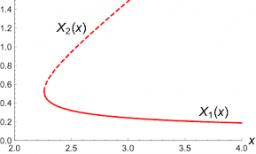# Increased 5906

The number z increased by 20 is 40% larger than the number z. Determine the number z.

z =  50

### Step-by-step explanation:

z+20 = 1.40 z

z+20 = 1.40•z

0.4z = 20

z = 50

Our simple equation calculator calculates it.Did you find an error or inaccuracy? Feel free to write us. Thank you!

Tips for related online calculators